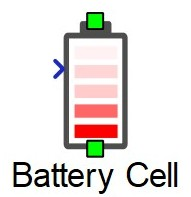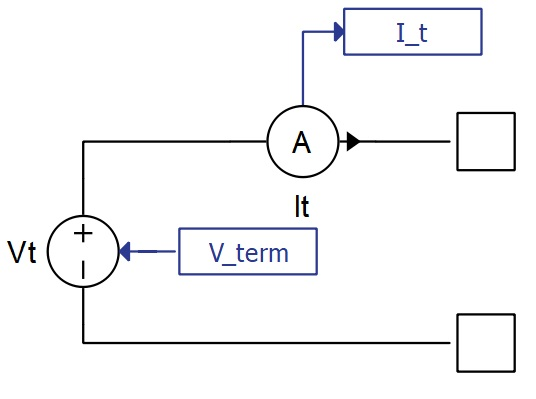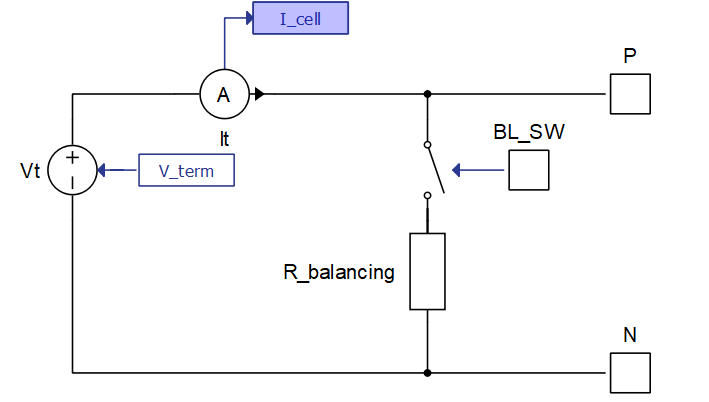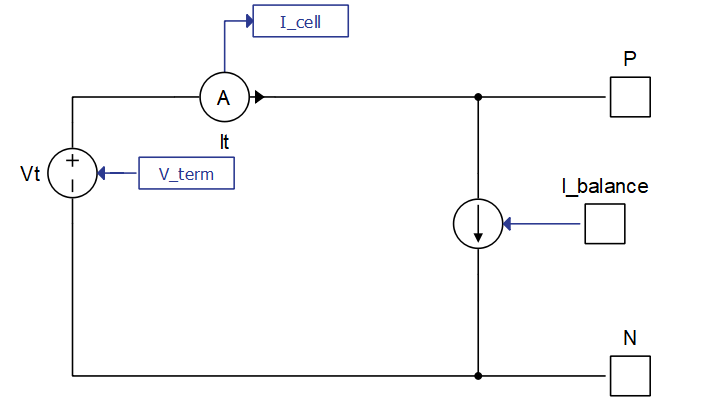# Battery Cell

Description of the Battery Cell component in Schematic Editor

Table 1. Battery Cell component in Schematic Editor
component component dialog window component parametersBattery Cell## Enhanced self correcting cell model.

The Battery Cell model is implemented in Typhoon HIL Schematic using a controlled voltage source and a current measurement. The terminal voltage signal that controls the voltage source is a signal processing function of previous states and the measured current, using the Enhanced self-correcting cell model.

The component has two power electronics terminals, with a positive terminal on top and a negative terminal on bottom of the component (as shown in the Battery Cell component icon). Additionaly, there is another signal processing input which can be either a scalar input of cells temperature, or a vector input of the cell temperature followed by a balancing input. This is covered in more detail in Tab: Basic parameters and Tab: Balancing circuit.

Short circuit of the cell can be simulated using the internal SCADA inputs of the component named Vcell Override and Vcell Value Set. When Vcell Override is set to 1, the terminal cell voltage of the component is bypassed and a new cell voltage matching the value of Vcell Value Set is assigned. Setting Vcell Override to 0 disables this feature.Figure 1. Schematic diagram of the Battery Cell model

Cell current is the current that is derived after subtracting the balancing current (if there is a balancing circuit enabled) from the total measured current (I_t in Figure 1).

The number of cells in parallel is modelled by dividing the cell current by the corresponding value in the parameter field. This method assumes that all the cells in the parallel configuration have equal parameters.

State of charge of the cell is calculated using the coulomb counting method with an adjustable coulombic efficiency coefficient:

Where $Q$ is the total capacity parameter, $i$ is the cell current, and $\eta$ is the coulombic efficiency coefficient, which is applied only when the cell is being charged (otherwise its value is set to 1).

The initial state of charge can be set inside the Initial state of charge property. Additionaly, state of charge can be overridden during the simulation by changing the State of Health SCADA input, SOH_set, inside of the Battery Cell component.

Terminal voltage (V_term in Figure 1) comprises four different components:
1. Open circuit voltage (OCV) which represents the cell's terminal voltage when the diffusion process has subsided, there is no current flow, and the hysteresis effect is ignored. It is always a function of state of charge and optionally a function of temperature.
2. Voltage drop on the internal resistance of the cell which is equal to the calculated internal battery cell resistance multiplied by the cell current (after balancing).
3. Voltage drop due to chemical diffusion which is the effect of ion diffusion of battery cells. It can be modeled as a variable number of parallel resistor capacitor circuits connected in series. The order of these resistor capacitor circuits can theoretically go to infinity, but in practice, up to a third order is often sufficient. During charging or discharging the battery cell, the capacitors are polarized and will require time to discharge themsleves when the current stops flowing through the battery.
4. Hysteresis effect voltage is important to replicate the state-of-the-art battery cell behavior. Combining all of the previous components of terminal voltage to accurately model the physical processes inside the battery cell is sometimes insufficient. When measuring the voltage on the terminals of the cell, hysteresis behavior of cell voltage in relation to cell current can be observed. One of the analytical equations that can be used to describe this behavior, called One state is described below:

Where:

,

M, M0, $\gamma$ are user customizable parameters, and $sig$ is a sign of the cell current.

## Tab: Basic parametersFigure 2. Basic parameters tab in the Battery Cell component

State of charge vector, Temperatures vector, and State of health vector are array-like elements and inputs into look up tables corresponding to other parameters inside this tab. State of charge vector is the only mandatory field out of these three for which at least one dimension of the Open circuit voltage vector look up table will be parametrized. Temperatures vector and State of health vector are evaluated only in the case that other parameters in this tab have multidimensional arrays as inputs. Temperature of the Battery Cell is provided by the signal processing input on the left side of this component.

Initial state of charge sets the starting state of charge of the battery cell component in percentages.

The Open circuit voltage vector parameter is an array-like element representing the data points corresponding to State of charge vector with an optional temperature dependance. For the model to compile, the length of State of charge vector must be equal to the number of columns in Open circuit voltage vector. If the parameter Open circuit voltage vector contains a two dimensional array-like element, then the rows must correspond to values inside the Temperatures vector parameter. In that case, columns are still data points of State of charge vector and must be of the same length. There must also be the same number of rows as the length of State of charge vector. The output of the OCV is the linear interpolation between the provided points corresponding to the current state of charge (and temperature if a two dimensional array-like element is provided).

The Nominal capacity combo box allows for parametrizing Battery Cell Total capacity by entering it directly or by calculating it from the available Discharge capacity. Choosing one option in this combo box will enable parameters corresponding to that option and disable parameters corresponding to the other option.
• Discharge capacity is the capacity that can be extracted from the fully charged battery with a particular constant current with a value of Discharge rate before it reaches the Minimum/cut-off voltage. Calculation is performed by including the effects of hysteresis (if enabled), diffusion voltage drop (if enabled), and the internal resistance voltage drop.
• Total capacity is the total amount of energy cell contains when fully charged. It is not possible to extract all this energy at any finite discharge current (it would take an infinite amount of time to extract it all), so cell capacities are not typically given in terms of maximum capacity, but it is a necessary parameter for State of Charge calculation.

The cell properties Total capacity (or Discharge capacity), Internal resistance, and Coulombic efficiency can each be constants, one dimensional array like elements, or two dimensional array-like elements. Using Internal resistance as an example, if a two dimensional array is provided, length of Temperatures vector must be equal to the number of columns in Internal resistance. There must also be the same number of rows as the length of State of health vector. If instead a one dimensional array is provided, the length of the Temperatures vector parameter will be the only dependance for calculating Internal resistance. A single value can also be provided for Internal resistance, in which case, no dependance is created and resistance is a constant value. The same applies for the Total capacity (or Discharge capacity) and Coulombic efficiency parameters.

Number of cells in parallel is an integer, positive number that represents the amount of identical cells to be paralleled in this battery cell.

Execution rate is the signal processing time step for calculating the cell terminal voltage based on previous states and the current input.

## Tab: Diffusion processFigure 3. Diffusion process tab in the Battery Cell component

Model order is a combo box which enables or disables up to three parallel connected resistor capacitor circuits. Values of resistors and capacitors are only applied if their respective properties are enabled.

• Resistor 1 parameter represents the resistor value in ohms for the first RC circuit
• Capacitor 1 parameter represents the capacitor value in farads for the first RC circuit
• Resistor 2 parameter represents the resistor value in ohms for the first RC circuit
• Capacitor 2parameter represents the capacitor value in farads for the first RC circuit
• Resistor 3 parameter represents the resistor value in ohms for the first RC circuit
• Capacitor 3 parameter represents the capacitor value in farads for the first RC circuit

## Tab: Voltage hysteresisFigure 4. Voltage hysteresis tab in the Battery Cell component

The Hysteresis model property allows you to select hysteresis effect implementation. Currently, only two implementations are allowed: None and One state.

If the One state option is selected, the Temperatures vector for hysteresis parameters and State of charge vector for hysteresis parameters properties are enabled and represent array-like data for two dimensional or one dimensional look up tables, depending on the M parameter, M0 parameter, and Gamma parameter fields.

Each of these parameters can be constants, one dimensional array-like elements, or two dimensional array-like elements. Using M parameter as an example, if a two dimensional array is provided, the length of Temperatures vector for hysteresis parameters must be equal to the number of columns in M parameter, and there must be the same number of rows as the length of State of charge vector for hysteresis parameters. If instead a one dimensional array is provided, the length of Temperatures vector for hysteresis parameters will be the only dependance for calculating M parameter. You can also provide a single value for M parameter, in which case no dependance is created and it is calculated as a constant value. The same applies for M0 parameter and Gamma parameter.

If None is selected for the Hysteresis model property, voltage from hysteresis is always set to zero.

## Tab: Balancing circuitFigure 5. Balancing circuit tab in the Battery Cell componentThe Balancing circuit combo box allows you to select any of the three following balancing circuits:
1. None: No balancing circuit is introduced and the cell current is the total terminal current.
2. Passive balancing: A new signal processing input is appended on the Battery Cell component signal processing input, making it a two dimensional (vectorized) signal input. The first component of this input remains the temperature signal, while the second input controls the BL_SW port of the balancing circuit. When the value of this second input is larger than 0.5, the ideal switch shown in Figure 6 is closed. In this case, the cell current used for calculating Battery Cell states and outputs is the remainder of the difference of the terminal input current and the current flowing through a balancing resistor. The value for the balancing resistor is provided in the property Balancing parallel resistor.Figure 6. Battery Cell model with passive balancing enabled
3. Direct input balancing: This option also modifies the signal processing input of the Battery Cell component into a two dimensional vector input. The second input signal is routed to the input port I_balance of the balancing circuit, shown in Figure 7. I_balance represents the value of the balancing current in amperes. In this case, the cell current used for calculating Battery Cell states and outputs is the remainder of the difference of the terminal input current and the input balancing current.Figure 7. Battery Cell model with direct input balancing enabled

## Tab: MeasurementsFigure 8. General tab in the Battery component
1. State of health checkbox enables/disables monitoring of Battery Cell state of health through a probe with the name SOH inside this component.
2. State of charge checkbox enables/disables monitoring of Battery Cell state of charge through a probe with the name SOC inside this component.
3. Open circuit voltage checkbox enables/disables monitoring of Battery Cell state of health through a probe with the name OCV inside this component.
4. Internal resistance checkbox enables/disables monitoring of Battery Cell state of health through a probe with the name Internal resistance inside this component.
5. Total capacity checkbox enables/disables monitoring of Battery Cell state of health through a probe with the name Total capacity inside this component.
6. Balancing current checkbox enables/disables monitoring of Battery Cell state of health through a probe with the name Balancing current inside this component.
7. Cell current checkbox enables/disables monitoring of Battery Cell state of health through a probe with the name Cell current inside this component.
8. Temperature checkbox enables/disables monitoring of Battery Cell state of health through a probe with the name Temperature inside this component.
9. Hysteresis voltage checkbox enables/disables monitoring of Battery Cell state of health through a probe with the name Hysteresis voltage inside this component.
Note: The Battery Cell component terminal current and voltage measurements are automatically enabled and can be monitored by selecting probes inside this component named It and Cell voltage.
Note: The Battery Cell component uses signal processing components. Disabling optional properties and providing constants or single dimensional data as parameters will reduce the signal processing computational load.